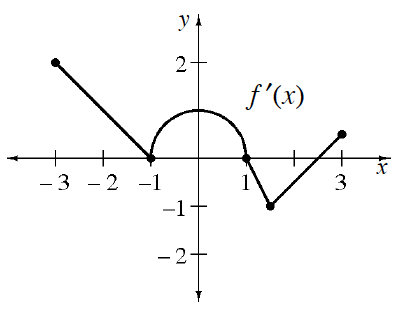### Home > APCALC > Chapter 5 > Lesson 5.4.1 > Problem5-141

5-141.

The graph below of $y = f ^\prime(x)$, the derivative of some function $f$, is composed of straight lines and a semicircle. Determine the values of $x$ for which $f$ has local minima, maxima, and points of inflection over the interval $[–3, 3]$.You are looking at the graph of $f '\left(x\right)$, but you are being asked to describe the graph of $f\left(x\right)$.

A minimum value on $f\left(x\right)$ is where the $y$-values change from decreasing to increasing. How does that show up on the $f '\left(x\right)$ graph?

Local minima on $f\left(x\right)$ are located anywhere that the graph of $f '\left(x\right)$ changes from negative to positive. Local maxima are the reverse.

Points of inflection on $f\left(x\right)$ occur where $f '\left(x\right)$ changes the sign of its slope. This happens twice.